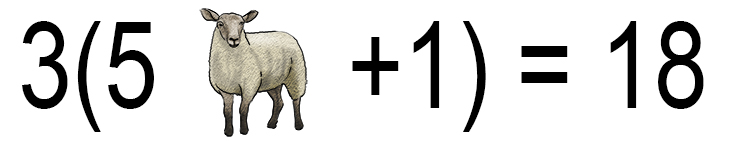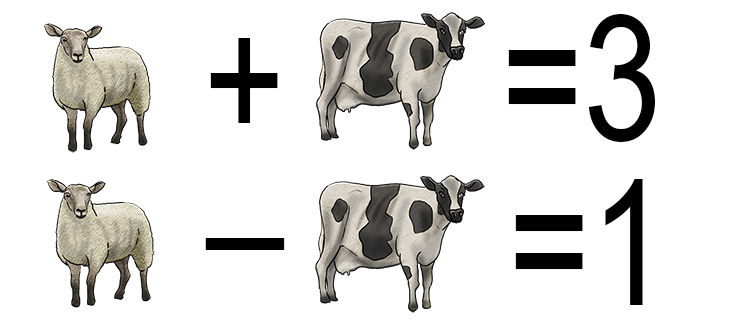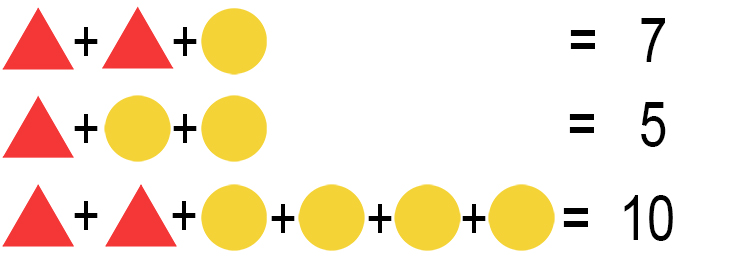# Fun with simultaneous equations

Example 1What is a sheep worth?

The way to tackle this question is to substitute the sheep for a letter. So the equation above becomes:

3(5x+1)=18

Multiply the 3 into the brackets:

3times5x+3times1=18

15x+3=18

Subtract 3 from both sides to help get x on its own:

15x+cancel(3)-cancel(3)=18-3

15x=15

Divide both sides by 15 to get x on its own:

(cancel(15)x)/cancel(15)=15/15

x=15/15

x=1

Answer: The sheep is worth 1.

Example 2What is a sheep worth and what is a cow worth?

The way to tackle this question is to substitute both the sheep and the cow with letters. So the equations become:

s+c=3 ...equation 1

s-c=1 ...equation 2

Take the first equation and subtract s from both sides to get c on its own:

s-s+c=3-s

c=3-s

Now substitute c into equation 2:

s-(3-s)=1

There is a 1 behind the minus sign but mathematicians usually hide it, but in this case we will put it back:

s-1(3-s)=1

Multiply the -1 into the brackets:

s-3+s=1

Add the two s's:

-3+2s=1

Add 3 to both sides to help get s on its own:

-cancel(3)+cancel(3)+2s=1+3

2s=4

Divide both sides by 2 to get s on its own:

(cancel(2)s)/cancel(2)=4/2

s=4/2

s=2

Now we know that s=2 we can plug that back into equation 1 or 2 to get the value of c.

Try equation 1:

s+c=3

2+c=3

Take 2 from both sides to get c on its own:

cancel(2)-cancel(2)+c=3-2

c=1

Answer: The sheep is worth 2 and the cow is worth 1.

Example 3What is the value of the question mark?

The way we tackle this question is to substitute each symbol for a letter i.e. sign = s, house = h, key = k.

h+h+h=15 ...equation 1

h+s+s=13 ...equation 2

s+k+k=8 ...equation 3

s+ktimesh=? ...equation 4

Immediately we can see that that equation 1 is the easiest to tackle:

h+h+h=15

Add the h's together and we get:

3h=15

Divide both sides by 3 to get h on its own:

(cancel(3)h)/cancel(3)=15/3

h=15/3

h=5

Substitute h=5 into equation 2 and we get:

h+s+s=13

5+s+s=13

5+2s=13

Minus 5 from both sides to help get s on its own:

cancel(5)-cancel(5)+2s=13-5

2s=8

Divide both sides by 2 to get s on its own:

(cancel(2)s)/cancel(2)=8/2

s=8/2

s=4

Substitute s=4 into equation 3 and we get:

s+k+k=8

4+k+k=8

4+2k=8

Minus 4 from both sides to help get k on its own:

cancel(4)-cancel(4)+2k=8-4

2k=4

Divide both sides by 2 to get k on its own:

(cancel(2)k)/cancel(2)=4/2

k=4/2

k=2

Substitute values of h=5, s=4 and k=2 into equation 4 and we get:

s+ktimesh=?

4+2times5=?

4+10=?

?=14

Answer: The value of the question mark is 14.

Example 4Find the value of a triangle and a circle.

The way to tackle this problem is to substitute each symbols for a letter i.e. triangle=x and circle= y.

x+x+y=7 ...equation 1

x+y+y=5 ...equation 2

x+x+y+y+y+y=10 ...equation 3

Which then becomes:

2x+y=7 ... equation 1

x+2y=5 ...equation 2

2x+4y=10 ...equation 3

We can see that equations 1 and 3 both have a 2x in them.

Tackling equation 1:

2x+y=7

Take y from either side to get 2x on its own:

2x+cancel(y)-cancel(y)=7-y

2x=7-y

Substitute this into equation 3:

2x+4y=10

7-y+4y=10

7+3y=10

Take 7 from both sides to help get y on its own:

cancel(7)-cancel(7)+3y=10-7

3y=3

Divide both sides by 3 to get y on its own:

(cancel(3)y)/cancel(3)=3/3

y=3/3

y=1

Substitute y=1 into equation 1 and we get:

2x+y=7

2x+1=7

Take 1 from both sides to help get x on its own:

2x+cancel(1)-cancel(1)=7-1

2x=6

Divide both sides by 2 to get x on its own:

(cancel(2)x)/cancel(2)=6/2

So y=1 and x=3.
or circle=1 and triangle =3.